﻿ NLVM 3 - 5 - Number & Operations Manipulatives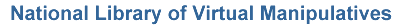Number & Operations (Grades 3 - 5)

Virtual manipulatives for Number & Operations, grades 3 - 5.Abacus – An electronic abacus that can be used to do arithmetic.Bar Chart – Create a bar chart showing quantities or percentages by labeling columns and clicking on values.Base Blocks – Illustrate addition and subtraction in a variety of bases.Base Blocks Addition – Use base ten blocks to model grouping in addition.Base Blocks Decimals – Add and subtract decimal values using base blocks.Base Blocks Subtraction – Use base ten blocks to model separation of groups in subtraction.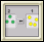Chip Abacus – Learn about carrying and digits using chips.Circle 0 – A puzzle involving adding positive and negative integers to sum to zero.Circle 21 – A puzzle involving adding positive and negative integers to sum to twenty one.Circle 3 – A puzzle involving adding positive real numbers to sum to three.Circle 99 – A puzzle involving adding positive and negative integers to sum to ninety nine.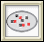Color Chips - Addition – Use color chips to illustrate addition of integers.Color Chips - Subtraction – Use color chips to illustrate subtraction of integers.Color Patterns – Arrange colors to complete a pattern.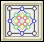Diffy – Solve an interesting puzzle involving the differences of given numbers.Factor Tree – Factor numbers using a tree diagram.Fraction Bars – Learn about fractions using fraction bars.Fraction Pieces – Work with parts and wholes to learn about fractions.Fractions - Adding – Illustrates what it means to find a common denominator and combine.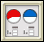Fractions - Comparing – Judge the size of fractions and plot them on a number line.Fractions - Equivalent – Illustrates relationships between equivalent fractions.Fractions - Naming – Write the fraction corresponding to the highlighted portion of a shape.Fractions - Parts of a Whole – Relates parts of a whole unit to written description and fraction.Fractions - Rectangle Multiplication – Visualize and practice multiplying fractions using an area representation.Fractions - Visualizing – Illustrate a fraction by dividing a shape and highlighting the appropriate parts.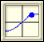Grapher – A tool for graphing and exploring functions.Hundreds Chart – Practice counting and visualize number patterns using a hundreds chart.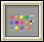Mastermind – Use inference and logic to play a game and guess a hidden pattern of pegs.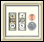Money – Learn about money by counting and making change.Number Line Arithmetic – Illustrates arithmetic operations using a number line.Number Line Bars – Use bars to show addition, subtraction, multiplication, and division on a number line.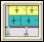Number Line Bars - Fractions – Divide fractions using number line bars.Number Line Bounce – Number line addition and subtraction game.Number Patterns – Discover the pattern and complete a sequence of numbers.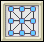Number Puzzles – Solve puzzles involving arranging numbers on a diagram so that they add up to a given value.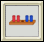Peg Puzzle – Win this game by moving the pegs on the left past the pegs on the right.Percent Grids – Represent, name, and explore percentages using hundreds grids.Percentages – Discover relationships between fractions, percents, and decimals.Pie Chart – Explore percentages and fractions using pie charts.Place Value Number Line – Explore place value by placing dots on number lines.Rectangle Division – Visualize and practice dividing numbers by using an area representation.Rectangle Multiplication – Visualize the multiplication of two numbers as an area.Rectangle Multiplication of Integers – Visualize and practice multiplying integers using an area representation.Sieve of Eratosthenes – Relate number patterns with visual patterns.Tangrams – Use all seven Chinese puzzle pieces to make shapes and solve problems.Venn Diagrams – Investigate common features of sets.